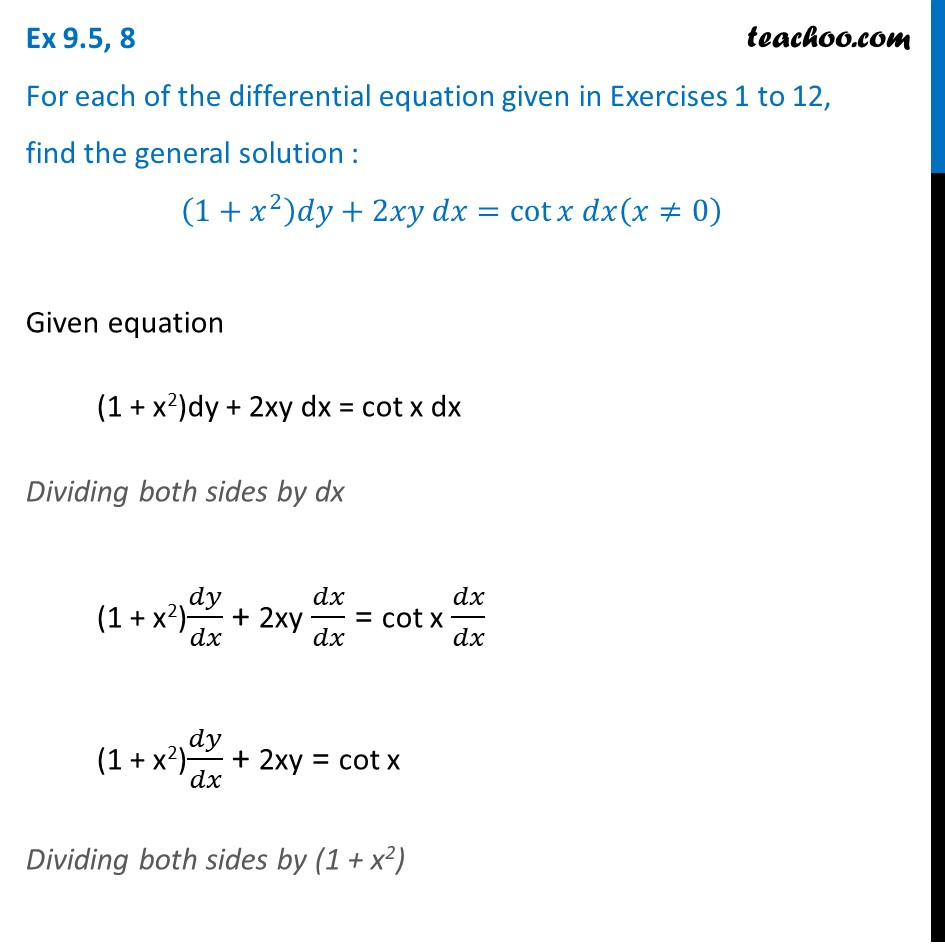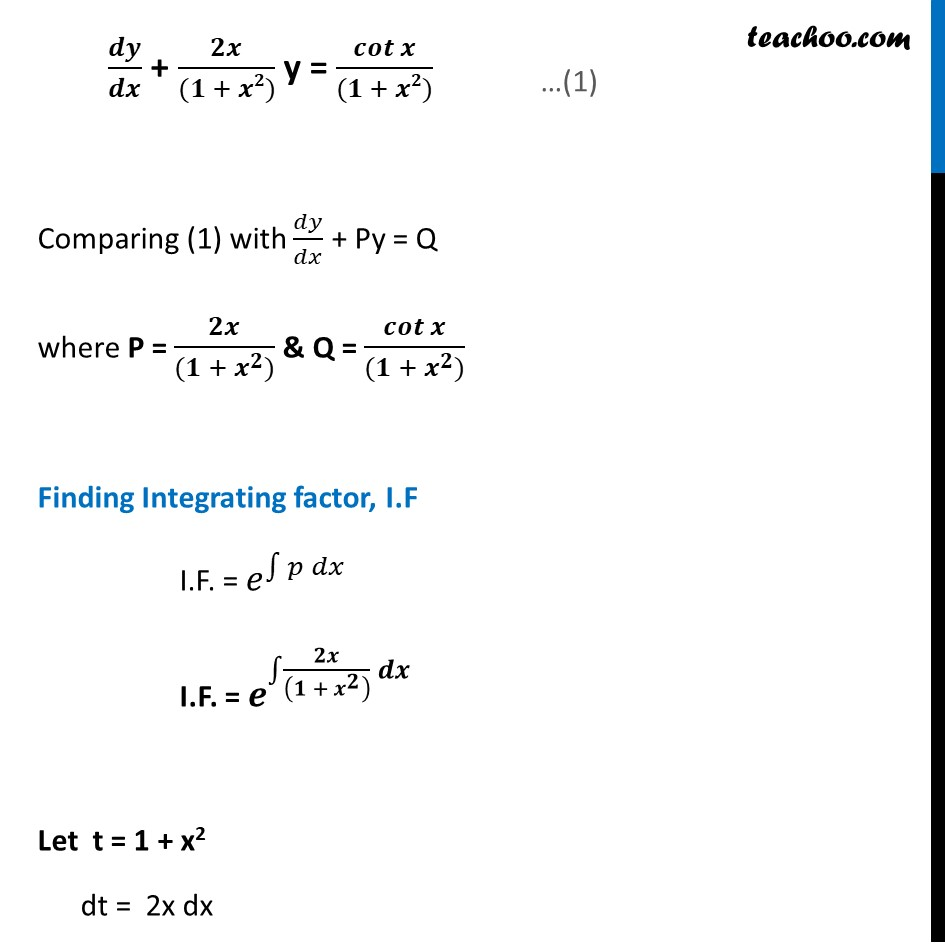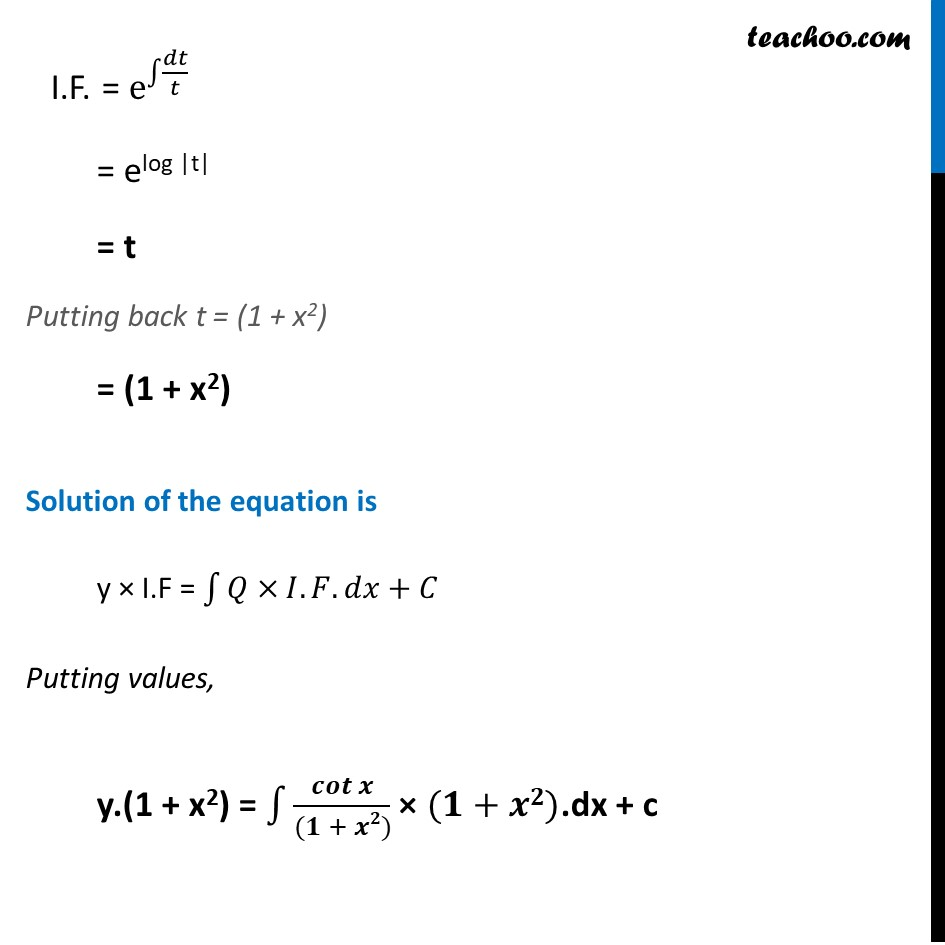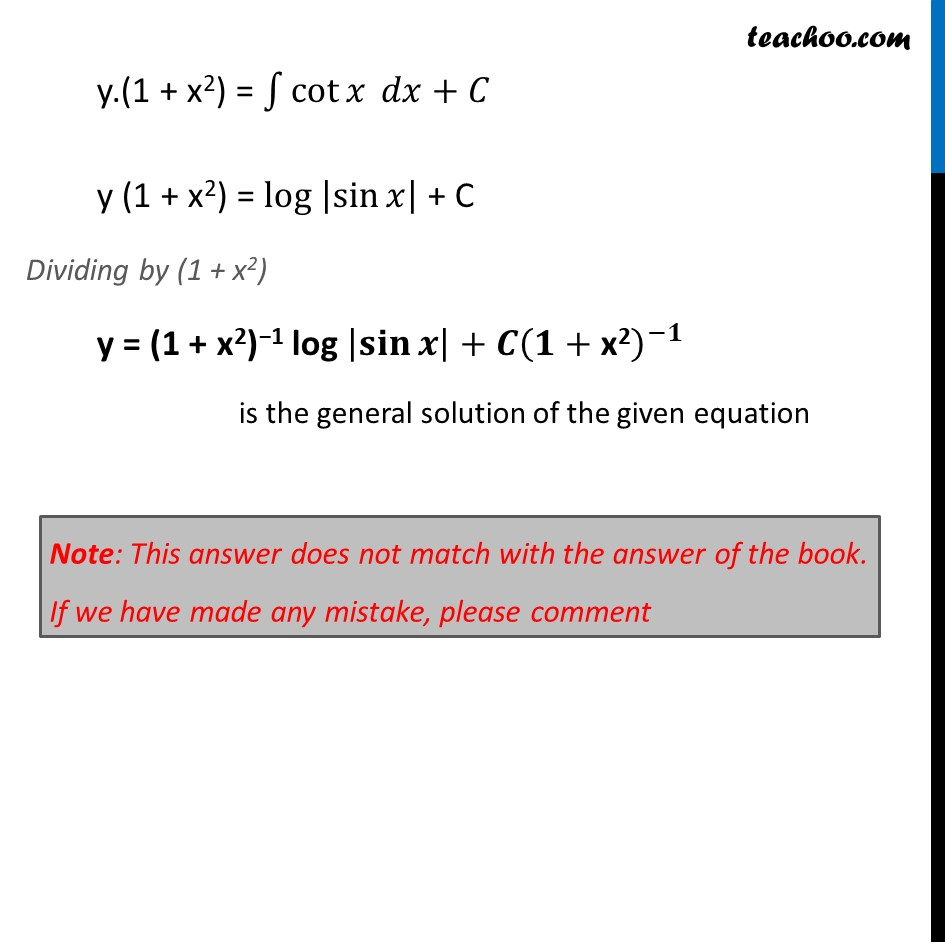Ex 9.5

Chapter 9 Class 12 Differential Equations
Serial order wiseLearn in your speed, with individual attention - Teachoo Maths 1-on-1 Class

### Transcript

Ex 9.5, 8 For each of the differential equation given in Exercises 1 to 12, find the general solution : (1+𝑥^2 )𝑑𝑦+2𝑥𝑦 𝑑𝑥=cot⁡〖𝑥 𝑑𝑥(𝑥≠0)〗 Given equation (1 + x2)dy + 2xy dx = cot x dx Dividing both sides by dx (1 + x2)𝑑𝑦/𝑑𝑥 + 2xy 𝑑𝑥/𝑑𝑥 = cot x 𝑑𝑥/𝑑𝑥 (1 + x2)𝑑𝑦/𝑑𝑥 + 2xy = cot x Dividing both sides by (1 + x2) 𝒅𝒚/𝒅𝒙 + 𝟐𝒙/((𝟏 + 𝒙𝟐)) y = 𝒄𝒐𝒕⁡𝒙/((𝟏 + 𝒙𝟐)) Comparing (1) with 𝑑𝑦/𝑑𝑥 + Py = Q where P = 𝟐𝒙/((𝟏 + 𝒙^𝟐)) & Q = 𝒄𝒐𝒕⁡𝒙/((𝟏 + 𝒙^𝟐)) Finding Integrating factor, I.F I.F. = 𝑒^∫1▒〖𝑝 𝑑𝑥〗 I.F. = 𝒆^∫1▒〖𝟐𝒙/((𝟏 + 𝒙^𝟐 ) ) 𝒅𝒙 〗 Let t = 1 + x2 dt = 2x dx I.F. = e^∫1▒〖𝑑𝑡/𝑡 〗 = elog |t| = t Putting back t = (1 + x2) = (1 + x2) Solution of the equation is y × I.F = ∫1▒〖𝑄×𝐼.𝐹.𝑑𝑥+𝐶〗 Putting values, y.(1 + x2) = ∫1▒𝒄𝒐𝒕⁡𝒙/((𝟏 + 𝒙𝟐)) × (𝟏+𝒙𝟐).dx + c y.(1 + x2) = ∫1▒〖cot⁡𝑥 𝑑𝑥〗+𝐶 y (1 + x2) = log |sin⁡𝑥 | + C Dividing by (1 + x2) y = (1 + x2)−1 log |𝐬𝐢𝐧⁡𝒙 |+𝑪(𝟏+"x2" )^(−𝟏) is the general solution of the given equation Note: This answer does not match with the answer of the book. If we have made any mistake, please comment